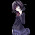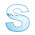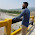Tuesday, July 21, 2015

Advanced Math Solutions – Limits Calculator, The Chain Rule

In our previous post, we talked about how to find the limit of a function using L'Hopital's rule. Another useful way to find the limit is the chain rule. When the chain rule comes to mind, we often think of the chain rule we use when deriving a function. However, the chain rule used to find the limit is different than the chain rule we use when deriving.

The Chain Rule:

What does the chain rule mean?

Given a function, f(g(x)), we set the inner function equal to g(x) and find the limit, b, as x approaches a. We then replace g(x) in f(g(x)) with u to get f(u). Using b, we find the limit, L, of f(u) as u approaches b. The limit of f(g(x)) as x approaches a is equal to L.

That sounds like a mouthful. Here we will go step by step for the first problem to better understand the chain rule (click here):

1. Find g(x) and f(u)

Since g(x) is the inner function, we set g(x)=\sin(x^2). We then replace the g(x) in f(g(x)) with u. Thus, f(u)=e^u.

2. Find the limit, b, of g(x)

3. Find the limit, L, of f(u)

Understanding the chain rule may be a little difficult, but once you practice some problems, which you can find on our website, the chain rule becomes much easier.

Until next time,

Leah

1.2.when ever i need calculation and want to know steps or procedure of aparticular problem ,i will remind symbolab it is a best math teACHER for me. i thanq all of them who developed nice calci and good website

3.What a Fabulous site
Always help me.......
No matter whatever the question is.....symbolab is there

4.I think that here you will find some info on how to write research paper and also some tips to write it better than your last one

5.Thanks for all the tips mentioned in this article! it’s always good to read things you have heard before and are implementing, but from a different perspective, always pick up some extra bits of information. [email protected]
mcafee.com/activate

mcafee.com/activate

norton.com/setup

6.